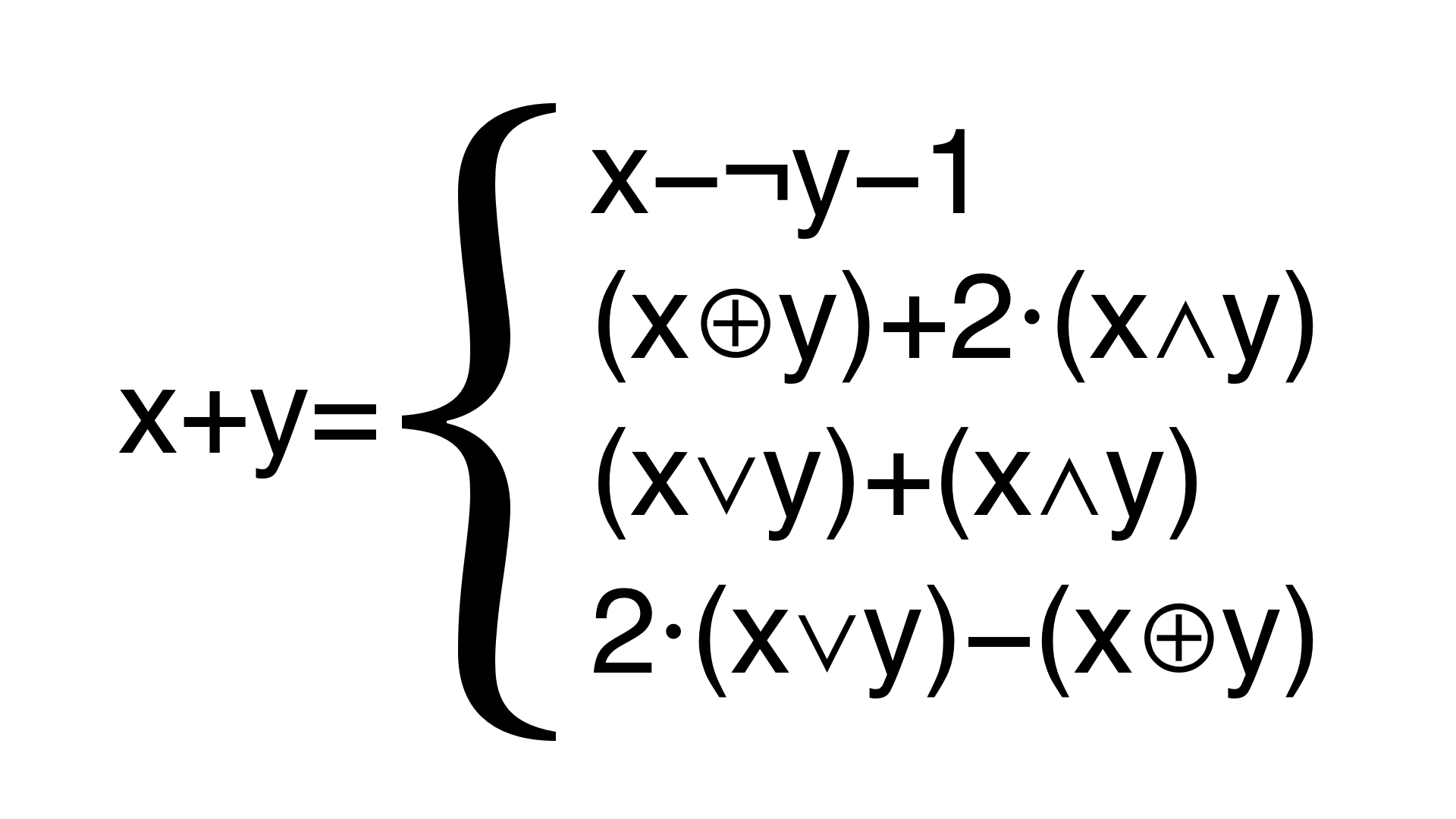# Encode Arithmeticfree web templates

Replace integer arithmetic with more complex expressions. For example, the following identities can be used to encode integer addition:

``````x + y = x - ¬ y - 1
= (x ⊕ y) + 2·(x ∧ y)
= (x ∨ y) + (x ∧ y)
= 2·(x ∨ y) - (x ⊕ y)
``````
free web templates

For example, Tigress might replace

``````z = x + y + w
``````
free web templates

with

``````z = (((x ^ y) + ((x & y) << 1)) | w) +
(((x ^ y) + ((x & y) << 1)) & w);
``````
OptionArgumentsDescription
--Transform EncodeArithmetic Replace integer arithmetic with more complex expressions.
--EncodeArithmeticKinds integer Specify the types to encode. Currently, only integer is available. Default=integer.
• integer = Replace integer arithmetic.
--EncodeArithmeticMaxLevel INTSPEC How deep to recurse into expressions. Default=100.
--EncodeArithmeticMaxTransforms INTSPEC How many transformations to perform on each expression. Default=100.
--EncodeArithmeticDumpFileName string Name of Json file onto which we dump transformed expression. The actual file will be function-name_number_fileName.json. From version 3.3.2. Default=100.

### Diversity

free web templates

For each operator, there are many possible encodings, and at transformation time, these are selected from randomly.

### Debugging and Attacking (From version 3.3.2

free web templates

There have been many recent papers on attacking MBA expressions. To facilitate such attacks you can dump all the transformed expressions onto a Json file for further processing. Simply set --EncodeArithmeticDumpFileName=filename.json.

### References

free web templates

Currently, the identities are taken from the book Hacker's Delight.